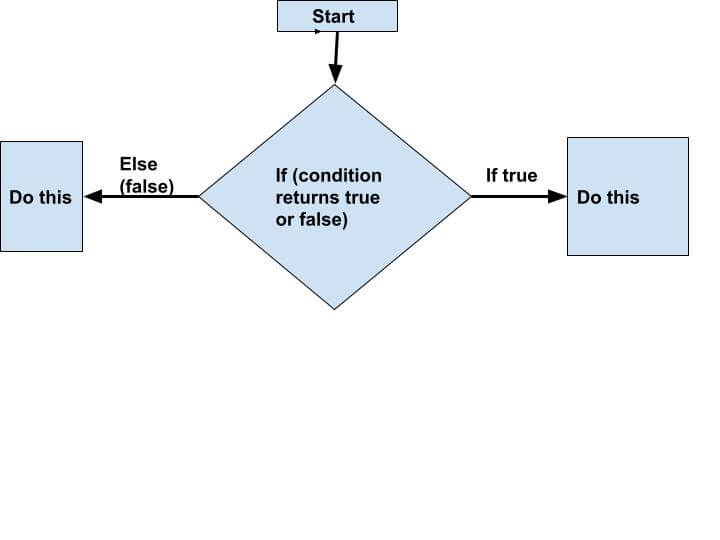Tutorials Hut

• # Scala if else and Conditions

Scala if else are conditions to check Boolean value (true/false) and accordingly execute the flow of the code. It is very similar to Java, C++ or any other programming language. Normally Scala programming discourages to use if else and prefers to do pattern matching by case.

See below diagram to understand flow of if else:## If Else Based Conditions

By using if and else keywords we can do branching in our code or logic, simple example of if else statement-

Example:

```object Conditions {

def main(args: Array[String]): Unit = {
//This is an example of if else where if part will execute
if(5 < 10) {
println("we are in if block, condition 5 < 10 is true")
}
else {
println("we are in else block, condition is false")
}

//This is an example of if else where else part will execute
if(15 < 10) {
println("we are in if block, condition is true")
}
else {
println("we are in else block, condition is 15 < 10 is false")
}
}
}

```

Output:

`we are in if block, condition 5 < 10 is truewe are in else block, condition is 15 < 10 is false`

## There are 3 different variations of if else:

·  If statement – We can simply use it if certain condition is true to do something

·  Else statement – We use this with if in case when if is not true and hence else bloc gets executed.

·  Else If statement – We can you this with an if conditions and when if is false code will come to this statement to check another condition and if this condition is true will execute the code.

## If Statement

If statement evaluates the condition and if true will execute the block belongs to if condition.

Syntax:

`if(condition which returns boolean) {  //Code to be execute if condition is true}`

Example:

```
object IfElseConditions {

def main(args: Array[String]): Unit = {

if(1<2) {
println("if condition is true 1<2 ")
}

if(12.3 < 100.9) {
println("if condition is true 12.3 < 100.9")
}
}
} ```

Output:

`if condition is true 1<2if condition is true 12.3 < 100.9`

## Else Statement

Else statement is always used with if or else if and if, if condition is false this will be executed.

Syntax:

`if(condition which returns false) {  //Code to be execute if condition is true}else {  //Code will execute if condition is false}`

Example:

```object IfElseConditions {

def main(args: Array[String]): Unit = {

if(10<2) {
println("if condition is true in if ")
}
else {
println("if was false, now in else for condition 10<2")
}
}
}```

Output:

if was false, now in else for condition 10<2

## Else If

Else if statement is always  used with any if or else if condition and when if condition is false this block will execute.

Syntax:

`if(condition which returns false) {  //Code to be execute if condition is true}else if (condition which returns false) {  //Code will execute if condition is false}`

Example:

```
object IfElseConditions {

def main(args: Array[String]): Unit = {

val a = 10;
if(a > 20) {
println("if condition is true, in if ")
}
else if(a > 5 ){
println("else if is true so in else if condition")
}
}
} ```

Output:

`else if is true so in else if condition`

• Scala Loop